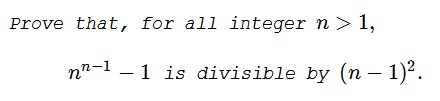# A Problem in Division of Polynomials

### Problem### Solution 1

We observe that, for $n\gt 1,$ the quotient of $n^{n-1}-1$ and $n-1$ is

$n^{n-2}+n^{n-3}+\ldots+1.$

We conceive this sum as resulting from the polynomial

$R(x)=(1+x)^{n-2}+(1+x)^{n-3}+\ldots+(1+x)+1$

when we substitute in it $x=n-1.$ In the expansion of $R(x)$ in powers of $x,$ the free term is $R(0)=n-1,$ and so

$R(x)=Q_{n-2}(x)x+(n-1),$

where $Q_{n-2}(x)$ is a polynomial of degree $n-3$ whose coefficients are integers. Now we substitute $n-1$ for $x$ and collect our conclusions:

\begin{align} n^{n-1}-1 &= (n-1)R(n-1)\\ &=(n-1)[Q_{n-2}(n-1)(n-1)+(n-1)]\\ &=(n-1)^2[Q_{n-2}(n-1)+1]. \end{align}

### Solution 2

Let $k=n-1$ with $k$ a positive integer. The property is trivially satisfied for $k=1$. For $k\gt 1$,

$(k+1)^k-1=k\left[1+(k+1)+(k+1)^2+...+(k+1)^{(k-1)}\right].$

Each term in the box bracket is congruent to $1$ modulo $k$ and there are $k$ such terms. Thus, the expression in the box bracket is divisible by $k$. Thus, $k^2|(k+1)^k-1$ or $(n-1)^2|n^{(n-1)}-1$.

### Solution 3

$\displaystyle \frac{n^{n-1}-1}{(n-1)^2}$ should result in an integer.

If it holds for $n$, it should hold for $n+1$. We replace $n$ by $n+1$, hence $\displaystyle f=\frac{(n+1)^n-1}{n^2}$ should give an integer.

We note that by the binomial theorem

\displaystyle\begin{align} (n+1)^n&=1+n^2+\frac{1}{2} (n-1) n^3\\ &\qquad\qquad+\frac{1}{6} (n-2) (n-1) n^4+\frac{1}{24} (n-3) (n-2) (n-1) n^5+\ldots \end{align}

which gives the desired result.

### Solution 4

With the change of variables $x = n-1$, we have

\displaystyle\begin{align} n^{n-1} - 1 &= (1+x) ^x -1\\ &=\left( \sum_{k=0}^x {{x} \choose{k}} x^{k} \right) - 1 & \text{Binomial Theorem}\\ &=\sum_{k=1}^x {{x} \choose{k}} x^{k} & {{x} \choose{0}} \; x^{0} = (1)(1)=1\\ &= x^2 + \sum_{k=2}^x {{x} \choose{k}} x^{k}. & {{x} \choose{1}} \; x^{1} = (x)(x)=x^2 \end{align}

Clearly, each term in the final expression is divisible by $x^2$.

This implies that $n^{n-1} -1$ is divisible by $(n-1)^2$, which is what we needed to demonstrate.

### Solution 5

\displaystyle \begin{align}n^{n-2} + \ldots + n^0 &\equiv 1^{n-2} + ... + 1^0\\ &= n-1 \equiv 0 \mod (n-1) \end{align}

so $n-1$ divides $\displaystyle n^{n-2} + \ldots + n^0 =\frac{n^{n-1}-1}{n-1}$.

### Solution 6

As in Solution 1,

$n^{n-1}-1=(n-1)(n^{n-2}+n^{n-3}+\ldots+1).$

By the long division algorithm,

\displaystyle \begin{align} n^{n-2}+n^{n-3}+\ldots+1&=(n-1)\left[\sum_{k=0}^{n-3}(n-2-k)n^k\right]-(n-1)]\\ &=(n-1)\left[\sum_{k=0}^{n-3}(n-2-k)n^k-1\right]. \end{align}

### Acknowledgment

The problem and Solution 1 came from The Stanford Mathematics Problem Book by G. Polya and J. Killpatrick (59.3). Solution 2 is by Amit Itagi; Solution 3 is by N. N. Taleb; Solution 4 is by Jim Henegan. Solution 5 came from anonymous comment.